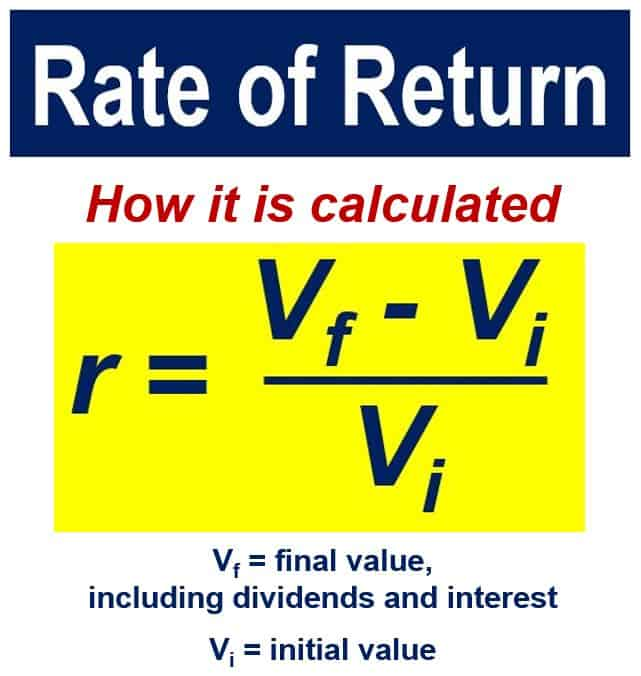# What is the rate of return (ROR)? Definition and meaning of ROR

The rate of return, ROR, or return, in the world of investments is the profit or loss you make on an investment. We usually express this as a yearly percentage.

Put simply; ROR is the ratio of the investment’s income over the cost of that investment. We use the rate of return as a measure of financial or economic success.If you had 200 shares, with a starting price of \$12, the starting value would be 200 x 12 = \$2,400. You then collected 0.50 per share in dividends, and the ending share price was 11.70, then at the end you would have 200 x 0.50 = \$100 in cash, plus 200 x 11.70 = \$2,340 in shares, totaling a final value of \$100 + \$2,340 = \$2,440. The change in value was \$2,400 minus \$2,340 = 60. So, the return was 60 ÷ 2000 = 3%.

You could calculate rate of return by expressing the economic gain, i.e., profit, as a percentage of the capital used to produce that gain.

We can also define it as the net amount of discounted cash flows obtained on an investment.

Any type of investment vehicle, including stocks, fine art, bonds, exchange-traded funds (EFTs), or real estate can yield a rate of return. It yields the return as long as that asset is bought at one point in time and produces cash flow at a future point in time.

When trying to determine which investments are the most attractive, we usually compare their past rates of return.

When you lose money on an investment, we call it a negative return.

According to lexicon.ft.com, the Financial Times’ glossary of terms, ‘rate of return’ is:

“The profit on an investment normally expressed as an annual percentage. This is typically the ratio of the income from the investment over the cost of the investment.”

If an investment has a ‘fair rate of return,’ it is worth the risk., i.e., it’s a worthwhile investment. The term also refers to the price limit that governments or regulatory agencies impose on utility companies.

## Return on equity vs. rate of return

##### Return on equity

This is a measure of how much cash a commercial enterprise can generate with each dollar of stockholder’s equity it receives. The term tells us how investor dollars have been and are being used.

We calculate return on equity (ROE) by taking a firm’s net income and dividing it by stockholder’s equity. Imagine John Doe Inc. generated \$10 million in net income over the course of one year. Its shareholder’s equity during that period was \$20 million.

In this case, the ROE would be 10 million ÷ 20 million = 50%. In other words, John Doe Inc. generated \$0.50 of profit for every stockholder dollar invested.

##### Rate of Return

The term refers to the loss or gain on an investment over a set period. I most cases, that period is one year. We can apply it to any type of investment, from mutual funds to stocks to bonds.

When deciding where to invest their money, savers and investors rely on the rate of return of different investment vehicles.

The most enticing investments are those with the highest historical rates of return.

## Rate of return of stocks vs. bondsWe calculate the rate of return of a stock and a bond differently. Imagine you bought a stock for \$50 per share, and after five years it earned you \$15 in dividends.

If you sold that share for \$90, you would have \$40 per share gain plus \$15 in income. The rate of return of that stock would be the total gain plus income (\$55). We then divide it by the \$50 cost per share, giving us a total of 110%.

Now imagine you paid \$2,000 for a \$2,000 par value 6% bond. The investment would earn you \$60 interest annually.

What if you sold the bond for \$2,200 and earned \$300 in total interest? Your rate of return would be the \$200 gain plus \$300 interest income. We then divide it by the \$2000 you paid for the bond, or 25%.

We use the IRR (internal rate of return) or ERR (economic rate of return) in capital budgeting to determine the expected growth rate of an investment.